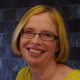14 May 2018
###### Numeracy crisis in New Zealand
16 June 2018

Introducing: the first post from Creative Maths. After seven years as Statistics Learning Centre, we have metamorphosed from the StatsLC caterpillar to a Creative Maths butterfly.  We hope you like our new look as much as we do. This better reflects our aim as a social enterprise to grow a world of mathematicians. We hope you can support us by using our materials, sponsoring our endeavours, and spreading the word.

In this post I explore further the idea of What Mathematicians do. You can find the first part here: What Mathematicians do, Part 1. It explains the purpose of the model and elaborates on two of the five sections – Mathematicians work in different ways, and Mathematicians strive.Mathematicians use a wide range of skills and behaviours

This graphic summarises the ideas of What mathematicians do. You can download a pdf of this from our free resources page.

In this post I will elaborate on Mathematicians explore, leaving Mathematicians reason, and Mathematicians seek solutions for a later post.

## Mathematicians explore

Much of the exploration that mathematicians do is in the world of abstract concepts. Sometimes this is dismissed as not practical, but who knows where the exploration can lead. Other exploration is initiated by problems in the physical or social world. In Königsberg, people wanted to work out a walking route that crossed all the bridges once each. Exploring this problem opened up a world of study, conjecture, analysis and wondering.

### Mathematicians notice and wonder

Curiosity is an essential characteristic of a mathematician. In our maths events we would notice that the red dragons seemed to be more likely to be dangerous. We would then wonder if it were really the case, or just something that happened in our sample. Noticing and wondering are important in statistics. We notice that honeycomb is always a regular hexagon pattern. We wonder if there is a mathematical reason for this (and it turns out that there is – clever bees!). We notice that multiples of three have digits that sum to multiples of three, and we wonder if this is the case in bases other than ten (and it turns out that it is related to the base.)

### Mathematicians find and make patterns

There are the obvious areas of pattern, such as tessellation and geometric reasoning. But then there are number patterns. For young children choral counting can open their eyes to the patterns in numbers. Pascal’s triangle produces a fascinating pattern, that occurs in multiple contexts. Some numbers can be represented in rectangular arrays, while others (primes over 13) can be expressed as sums of two rectangular arrays.

### Mathematicians use multiple representations

Recently I discovered Graph Theory, an exciting area of mathematics. I am embarrassed to admit that I managed to study and teach Operations Research to a high level without realising that Graph Theory existed. One of the amazing things is that it uses different representations to explore ideas. Depending on how we represent a problem, we get different insights. Similarly, in probability we can use a Venn diagram, a tree or a table to represent the same idea, and gain different insights.

### Mathematicians play with ideas

Mathematics is all about ideas. What is infinity? Are there different kinds of infinity? Are some infinities bigger than others? Is it possible to draw a pattern that requires more than four colours to colour it, with no two touching shapes the same colour? Are there patterns in the way that prime numbers appear?

### Mathematicians experiment and create

As operations researchers, simulations and models were our stock in trade. We try out ideas, we make models and see what happens when we tweak parameters. Spreadsheets enable a wider range of experimentation and sometimes enumeration. Our new identity as Creative Maths reflects our desire to create things. We create videos, games and learning activities that help people to understand mathematical concepts and embrace mathematics.

### Mathematicians have fun

Recently I asked about a hundred people, including primary teachers and secondary maths teachers, to list six words that they would use to describe mathematics. The most common word was fun! Even some of the people who described their feelings about maths as negative said that maths was fun.

For me, the fun of mathematics is in the exploring, and the challenge and the failure and success. All over the world people play puzzles like Sudoku. I choose the difficult ones, and love the way my brain strains, and the feeling of satisfaction when I nail it! Similarly, if we are trying to solve a mathematical problem, it can be immensely satisfying to make some progress. When you work with another mathematician on a problem, it can be even more fun to build on each other’s ideas and findings.

## This matters

In New Zealand and much of the English-speaking world, there is a negative perception of mathematics as an optional skill – more like playing the piano than like reading and writing. Yet mathematics attainment is used as a filter to higher education, which can be a filter to higher pay and power. We need all of our citizens to embrace mathematics and perceive it as necessary, enjoyable and attainable. Reframing our definition of what mathematicians do is a step towards a world of mathematicians.##### Dr Nic

1.Rebecca says:
2.Dr Nic says: